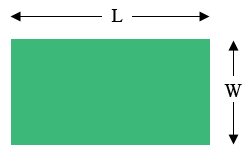SEARCH HOMEMath Central Quandaries & QueriesQuestion from Chie: A rectangular piece of property has an area of 987m² and a perimeter of 136m. Find its LENGTH and WIDTH.Hi,

Suppose the length is $L$ meters and the width is $W$ meters. Since the area is $987 m^2$ you know that

$L \times W = 987.$

Since the perimeter is 136 m you know that the distance all the way around is 136 m and hence the distance half the way around is 68 m.This gives a second equation

$L + W = 68.$

Solve the two equations for $L$ and $W.$

PennyMath Central is supported by the University of Regina and The Pacific Institute for the Mathematical Sciences.